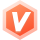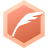# 每日一刷：力扣828. 统计子串中的唯一字符知识浅谈 🐄🐄🐄🐄🐄大佬后端领域优质创作者博客专家认证
2022-09-06 14:38:54

``````class Solution {
public int uniqueLetterString(String s) {
int index,sum=0;
char[] chs = s.toCharArray();
int len = chs.length;
int l,r;
for(char c='A';c<='Z';c++){
l=r=-1;
for(int i=0;i<len;i++){
if(chs[i]==c){
l=r;r=i;
}
sum+=(r-l);
}
}
return sum;
}
}``````

...全文
8 回复 打赏 收藏 举报力扣 828. 统计子串唯一字符力扣828. 统计子串唯一字符LeetCode 828. 统计子串唯一字符心扩展）Leetcode 828. 统计子串唯一字符 C++828. 统计子串唯一字符

1840

java爬虫后端 个人社区 吉林省·长春市

【社区倡导与禁止】

• 社区倡导积极发帖，互相交流技术问题，学习心得。
• 社区不限制技术领域，后端内容增加加精权重
• 社区禁止发布低俗、不健康的内容

【社区积分规则】

• 在社区「发帖」得10积分
• 内容被管理员「加精」得10积分
• 点赞他人内容得1积分
• 评论内容得2积分